# Python数据分析之Pandas2018年4月27日06:04:32

4 21947字阅读73分9秒

Pandas 建立在 NumPy 基础之上，但增加了更加高级实用的功能，比如数据自动对齐功能，时间序列的支持，缺失数据的灵活处理等等。这里我们就会介绍一下 Pandas 的简单的使用。

## Pandas介绍

1. import numpy as np
2. import pandas as pd
3. from pandas import Series,DataFrame

## Series和DataFrame

`Series``DataFrame``Pandas`中的两种核心数据结构，大部分`Pandas`的功能都围绕着两种数据结构进行。下面分别对这两个数据结构进行介绍.

## Series介绍

### 创建Series数据

`Series`是值的序列，可以理解为一维数组，它只有一个列和索引。索引可以定制，当不指定时默认使用整数索引，而且索引可以被命名:

1. s1 = Series([1,2,3,4,5])
2. >>
3. 0    1
4. 1    2
5. 2    3
6. 3    4
7. 4    5
8. dtype: int64

``````s1 = Series([1,2,3,4,5],index=['a','b','c','d','e'])
s1.index.name = 'index'
>>
index
a    1
b    2
c    3
d    4
e    5
dtype: int64``````

### Series的选择

``````s1 = Series([1,2,3,4,5],index=['a','b','c','d','e'])
>>
a    1
b    2
c    3
d    4
e    5
dtype: int64

s1
>> 1
s1['a']
>> 1

s1[1:3]
>>
b    2
c    3
dtype: int64

s1['b':'c']
>>
b    2
c    3
dtype: int64``````

``````s1.iloc[1:3]
>>
b    2
c    3
dtype: int64

s1.loc['b':'c']
>>
b    2
c    3
dtype: int64``````

### 使用滑动窗口-移动平均值计算

• window，Size of the moving window. This is the number of observations used for calculating the statistic. Each window will be a fixed size.
• min_periods，Minimum number of observations in window required to have a value (otherwise result is NA)
• win_type，Provide a window type. If `None`, all points are evenly weighted.

1. df = pd.DataFrame({'B': [0, 1, 2, 3, 4]})
2. """
3.    B
4. 0  0
5. 1  1
6. 2  2
7. 3  3
8. 4  4
9. """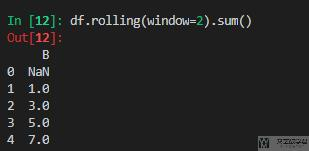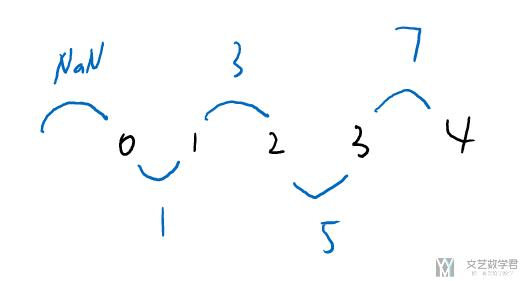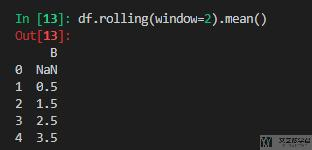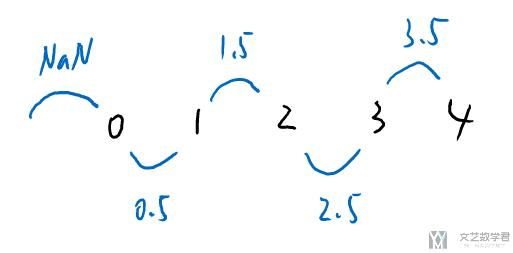## DataFrame介绍

### 创建DataFrame数据

`DataFrame`类似于二维数组，有行和列之分，除了像`Series`一样，多个行有索引而外，每个列上面还可以有标签`label`, 索引和标签本身都可以被命名：

1. df = DataFrame(np.random.random((5,4)),index=['a','b','c','d','e'],columns=['A','B','C','D'])
2. >>
3.           A         B         C         D
4. a  0.095161  0.982524  0.253735  0.105706
5. b  0.795408  0.727552  0.183551  0.565994
6. c  0.968957  0.283453  0.630097  0.308991
7. d  0.310784  0.677971  0.417679  0.793429
8. e  0.042881  0.817054  0.508455  0.914367

1. x_test = np.array([1,2,3])
2. y_test = np.array([,,])

1. pdd = pd.DataFrame(x_test, columns=['x'])
2. pdd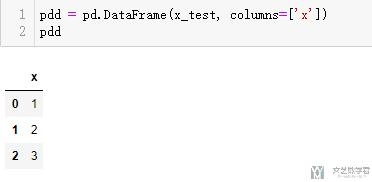1. pdd['y'] = y_test
2. pdd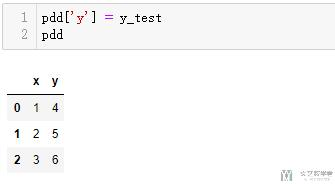### 按行获取 Dataframe 的信息

1. import pandas as pd
2. df = pd.DataFrame({'c1': [10, 11, 12], 'c2': [100, 110, 120]})
3. for index, row in df.iterrows():
4.     print('行', index, row['c1'], row['c2'])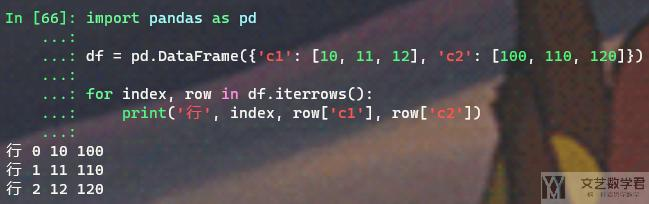### Dataframe 完整显示

1. def print_full(x):
2.     pd.set_option('display.max_rows', None)
3.     pd.set_option('display.max_columns', None)
4.     pd.set_option('display.width', 2000)
5.     pd.set_option('display.float_format', '{:20,.2f}'.format)
6.     pd.set_option('display.max_colwidth', None)
7.     print(x)
8.     pd.reset_option('display.max_rows')
9.     pd.reset_option('display.max_columns')
10.     pd.reset_option('display.width')
11.     pd.reset_option('display.float_format')
12.     pd.reset_option('display.max_colwidth')

1. pd.set_option('display.max_rows', None)
2. pd.set_option('display.max_columns', None)
3. pd.set_option('display.width', 2000)
4. pd.set_option('display.max_colwidth', None)

### Dataframe 的显示-在 jupyter notebook 显示

1. table = pd.DataFrame(np.zeros((3, 2)))
2. print(table)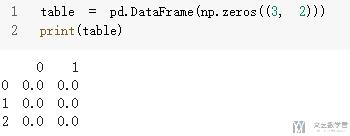1. from IPython import display
2. from IPython.display import HTML # 引入 display 模块目的方便程序运行展示

1. html = table.to_html()
2. display.display(HTML(html))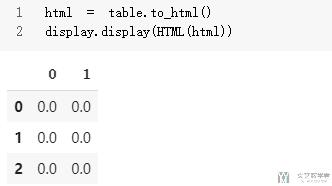### Dataframe 的显示-在文件中打印

1. from tabulate import tabulate
2. import pandas as pd
3. df = pd.DataFrame({'col_two' : [0.0001, 1e-005 , 1e-006, 1e-007],
4.                    'column_3' : ['ABCD', 'ABCD', 'long string', 'ABCD']})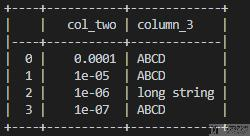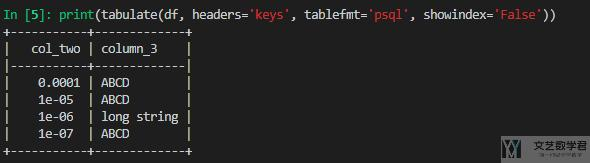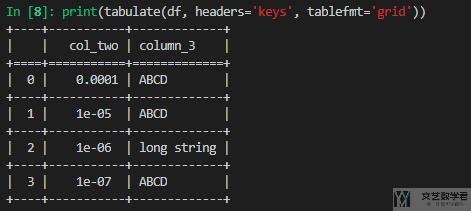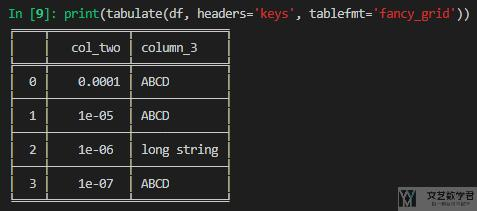### Dataframe重命名-rename

1. test = pd.DataFrame([[1,2,3],[4,5,6]])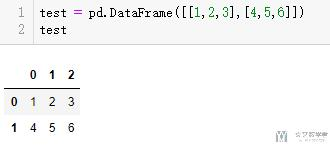1. test.rename(columns={0:'A',1:'B',2:'C'}, inplace=True)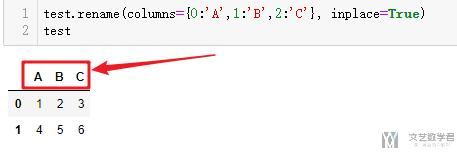1. test.rename(index={0:'C1',1:'C2'}, inplace=True)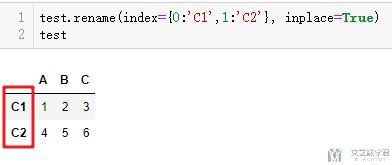### 直接对原始变量修改-inplace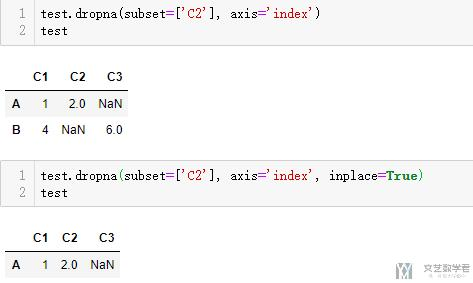### Dataframe删除行或列-drop

1. test = pd.DataFrame([[1,2,3],[4,5,6]], index=['A','B'], columns=['C1','C2','C3'])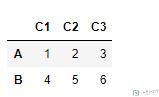1. test.drop(['A'], axis=0) # 删除行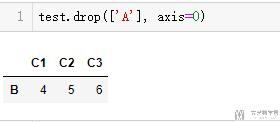1. test.drop(['C2','C3'], axis=1) # 删除列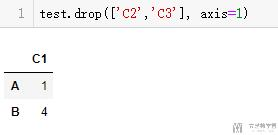### 选择 Dataframe 元素

``````df = DataFrame(np.random.random((5,4)),index=['a','b','c','d','e'],columns=['A','B','C','D'])
>>
A         B         C         D
a  0.798929  0.987169  0.495320  0.623631
b  0.000925  0.335466  0.117363  0.007925
c  0.730285  0.616586  0.972650  0.503028
d  0.783396  0.246152  0.084311  0.977647
e  0.105396  0.123413  0.284357  0.292990

df['A'] #获取一列的数据
>>
a    0.798929
b    0.000925
c    0.730285
d    0.783396
e    0.105396
Name: A, dtype: float64

df[df.columns[0:3]] #获取多列数据
>>
A         B         C
a  0.798929  0.987169  0.495320
b  0.000925  0.335466  0.117363
c  0.730285  0.616586  0.972650
d  0.783396  0.246152  0.084311
e  0.105396  0.123413  0.284357

"""

"""

df.columns
>> Index(['A', 'B', 'C', 'D'], dtype='object')``````

1. i[['query_id','query_title_id']]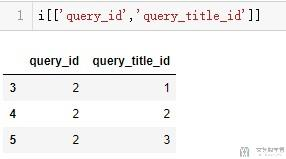1. i.loc[:,['query_id','query_title_id']]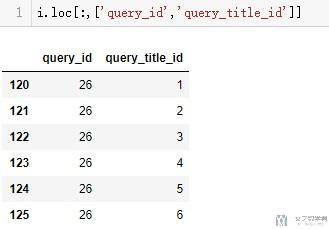``````df.loc['a'] #选择一行数据
>>
A    0.798929
B    0.987169
C    0.495320
D    0.623631
Name: a, dtype: float64

df.loc['a':'c'] #选择多行数据
>>
A         B         C         D
a  0.798929  0.987169  0.495320  0.623631
b  0.000925  0.335466  0.117363  0.007925
c  0.730285  0.616586  0.972650  0.503028``````

``````df.loc['a','A']
>> 0.79892857875107204

df.loc['a':'c','A':'C']
>>
A         B         C
a  0.798929  0.987169  0.495320
b  0.000925  0.335466  0.117363
c  0.730285  0.616586  0.972650``````

### 对dataframe内元素排序-sort_values

1. test = pd.DataFrame([[1,2],[3,4],[2,1]], index=['A','B','C'], columns=['C1','C2'])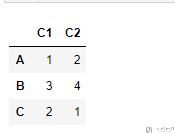1. test.sort_values(by=['C1'], ascending=True)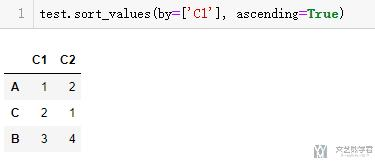### 重新排序index

1.  # index重新排序
2. test = pd.DataFrame(np.array([[1,2],[3,4],[5,6],[7,8]]),columns=['a','b'])
3. print("原始数据\n{}".format(test))
4. test = test.sample(frac=1)
5. print("重新采样数据\n{}".format(test))
6. test.reset_index(drop=True, inplace=True)
7. print("Reset Index后\n{}".format(test))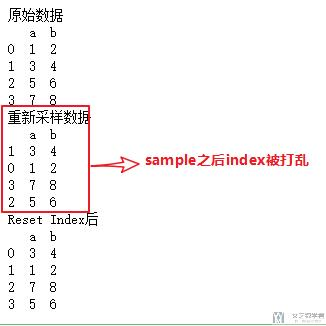## 缺失值和数据自动对齐

`Pandas`中最重要的一个功能是，它可以对不同索引的对象进行算术运算。比如将两个`Series`数据进行相加时，如果存在不同的索引，则结果是两个索引的并集，什么意思呢？通过例子看下：

``````s1 = Series([1, 2, 3, 4], index=['a', 'b', 'c', 'd'])
>>
a    1
b    2
c    3
d    4
dtype: int64

s2 = Series([2, 3, 4, 5], index=['b', 'c', 'd', 'e'])
>>
b    2
c    3
d    4
e    5
dtype: int64

s1+s2
>>
a    NaN
b    4.0
c    6.0
d    8.0
e    NaN
dtype: float64``````

``````df1 = DataFrame(np.arange(9).reshape(3,3), columns=list('ABC'), index=list('abc'))
>>
A  B  C
a  0  1  2
b  3  4  5
c  6  7  8

df2 = DataFrame(np.arange(12).reshape(3,4),columns=list('ABDE'), index=list('bcd'))
>>
A  B   D   E
b  0  1   2   3
c  4  5   6   7
d  8  9  10  11

df1+df2
>>
A     B   C   D   E
a   NaN   NaN NaN NaN NaN
b   3.0   5.0 NaN NaN NaN
c  10.0  12.0 NaN NaN NaN
d   NaN   NaN NaN NaN NaN``````

``````df1.add(df2,fill_value=0)
>>
A     B    C     D     E
a   0.0   1.0  2.0   NaN   NaN
b   3.0   5.0  5.0   2.0   3.0
c  10.0  12.0  8.0   6.0   7.0
d   8.0   9.0  NaN  10.0  11.0``````

### 缺失内容的删除-dropna

1. test = pd.DataFrame([[1,2,3],[4,np.nan,6]], index=['A','B'], columns=['C1','C2','C3'])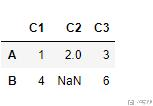1. test.dropna(axis='index') # 按行删除
2. test.dropna(axis='columns') # 按列删除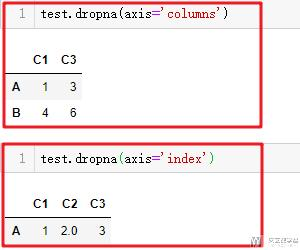1. # 下面两种写法是一样的
2. test.dropna(subset=['C2'], axis='index')
3. test.dropna(subset=['C2'])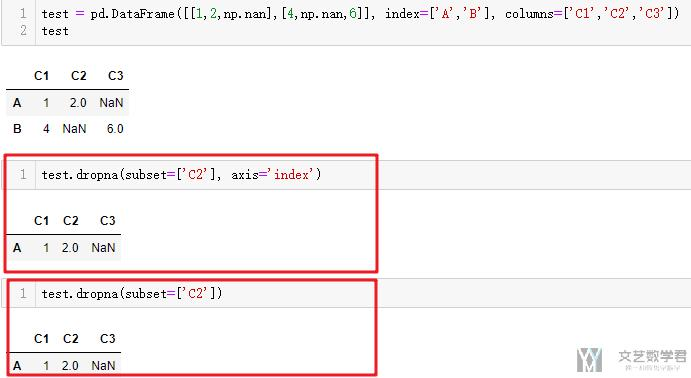## 常用统计

``````df1 = DataFrame(np.arange(9).reshape(3,3), columns=list('ABC'), index=list('abc'))

df1.describe()
>>
A    B    C
count  3.0  3.0  3.0
mean   3.0  4.0  5.0
std    3.0  3.0  3.0
min    0.0  1.0  2.0
25%    1.5  2.5  3.5
50%    3.0  4.0  5.0
75%    4.5  5.5  6.5
max    6.0  7.0  8.0``````

• `count` 元素值的数量；
• `mean` 平均值；
• `std` 标准差；
• `min` 最小值；
• `25%` 下四分位数；
• `50%` 中位数；
• `75%` 上四分位数；
• `max` 最大值；

### 按行或列进行运算

``````df1 = DataFrame(np.arange(9).reshape(3,3), columns=list('ABC'), index=list('abc'))
>>
A  B  C
a  0  1  2
b  3  4  5
c  6  7  8

f = lambda x: x.max() - x.min() #这里也可以不使用匿名函数

def f(x):
return x.max()-x.min()

df1.apply(f)
>>
A    6
B    6
C    6
dtype: int64

df1.apply(f,axis=1)
>>
a    2
b    2
c    2
dtype: int64``````

## 数组的变形

### Melt-dataframe变形

melt是将宽表变为长表, 我自己感觉melt还是很好用的. 我们下面看一个例子, 比如有如下形式的数据.

1. df = pd.DataFrame(([1,2,3,4,5,6],[7,8,9,10,11,12],[13,14,15,16,17,18]),
2.                   index=['A','B','C'],
3.                   columns=['Pop', 'GDP', '1/2', '1/3', '1/4', '1/5'])
4. df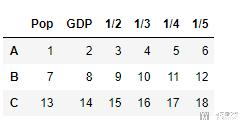1. pd.melt(df, id_vars=['Pop','GDP'], var_name = 'date', value_name='Confirmed')

• id_vars: 表示不展开的列, 最后是保留的变量
• var_name: 变量的名字, 在这里我们是要将1/2, 1/3展开, 这里都是日期.
• value_name: 这个是展开之后数字的名称.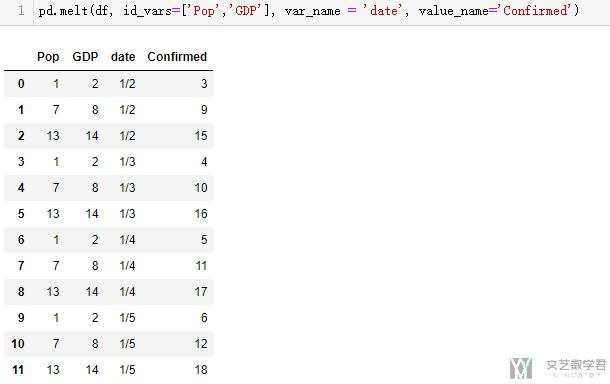### Pivot-透视表

1. pd.pivot(test, index='Name', columns='date', values='Confirmed')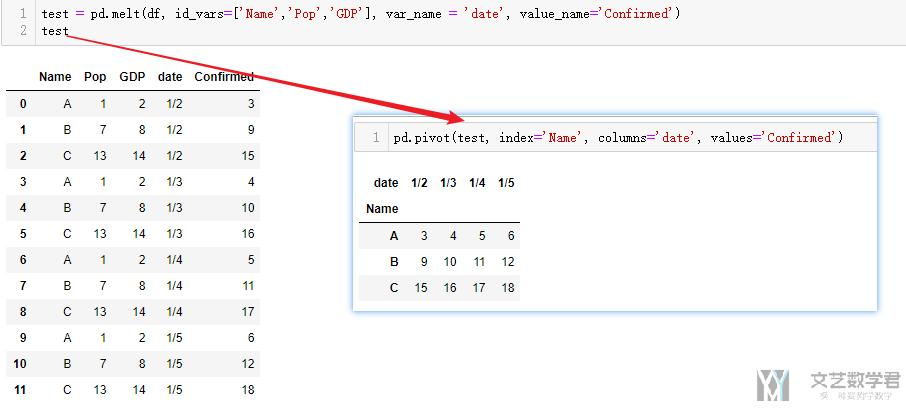### Stack与Unstack-01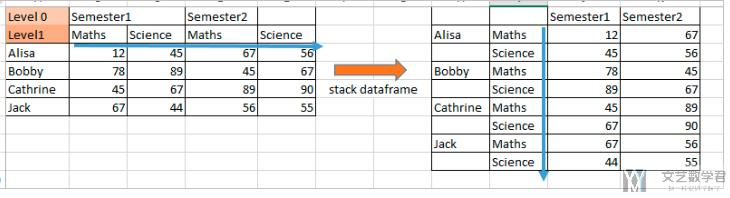2. d=([[12,45,67,56],[78,89,45,67],[45,67,89,90],[67,44,56,55]])
3. df = pd.DataFrame(d,
4.                   index=['Alisa','Bobby','Cathrine','Jack'],
6. df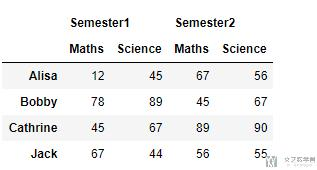1. df.stack(level=1)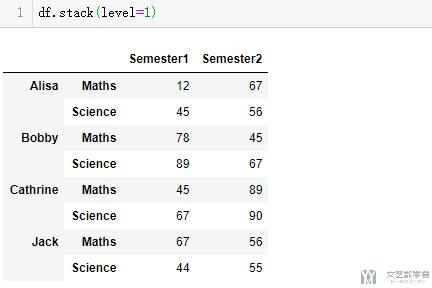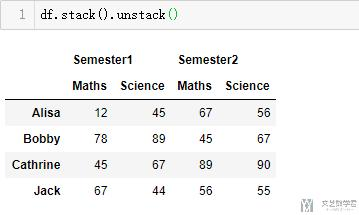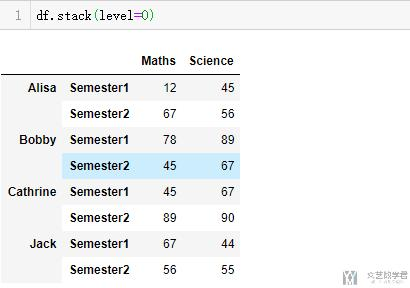### Stack与Unstack-02

stack除了上面的情况外, 我们更多的是见到一行列标题的表, 此时stack的作用就是将宽表变为长表. 我们看下面这个例子.

1. df = pd.DataFrame(([1,2],[3,4],[5,6]), index=['A','B','C'], columns=['1/2','1/3'])
2. df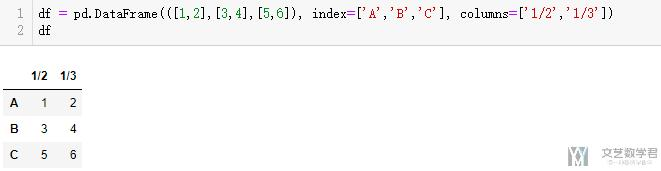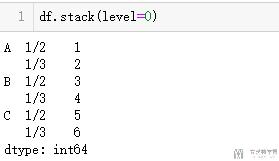## 数据合并和分组

### 数组合并-concat

1. df1 = DataFrame(np.random.randn(3, 3))
2. >>
3.           0         1         2
4. 0  0.506929  0.482098  0.142396
5. 1 -1.431190  0.527396  0.784056
6. 2 -1.184716  2.082178  0.666990
7. df2 = DataFrame(np.random.randn(3, 3), index=[5, 6, 7])
8. >>
9.           0         1         2
10. 5  0.611993 -0.264532 -0.022721
11. 6  0.436712 -0.365940  1.430864
12. 7 -0.646884  0.368955 -0.704303
13. pd.concat([df1,df2])
14. >>
15.           0         1         2
16. 0  0.506929  0.482098  0.142396
17. 1 -1.431190  0.527396  0.784056
18. 2 -1.184716  2.082178  0.666990
19. 5  0.611993 -0.264532 -0.022721
20. 6  0.436712 -0.365940  1.430864
21. 7 -0.646884  0.368955 -0.704303

### 数组的添加-append

1. df1 = pd.DataFrame(np.random.randn(3, 3))
2. df1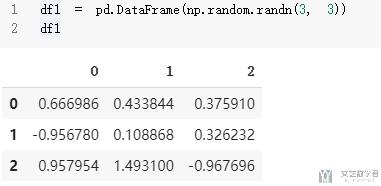1. df2 = pd.Series([np.NAN]*3, index=df1.columns, name=str(['Append']))
2. df2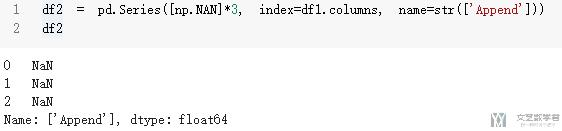1. df1.append(df2)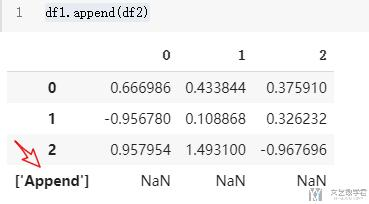### 数组的合并-merge

1. df1 = DataFrame({'user_id': [5348, 13], 'course': [12, 45], 'minutes': [9, 36]})
2. >>
3.    course  minutes  user_id
4. 0      12        9     5348
5. 1      45       36       13
6. df2 = DataFrame({'course': [12, 45], 'name': ['Linux 基础入门', '数据分析']})
7. >>
8.    course        name
9. 0      12  Linux 基础入门
10. 1      45        数据分析
11. pd.merge(df1,df2)
12. >>
13.    course  minutes  user_id        name
14. 0      12        9     5348  Linux 基础入门
15. 1      45       36       13        数据分析

1. inputData = pd.merge(inputData,tf_idf_group_datafram,on=['query_id','query_title_id'])

#### 多个dataframe的merge

1. left = pd.DataFrame({'key': ['K0', 'K1', 'K2', 'K3'],
2.                      'A': ['A0', 'A1', 'A2', 'A3'],
3.                      'B': ['B0', 'B1', 'B2', 'B3']})
4. middle = pd.DataFrame({'key': ['K0', 'K1', 'K2', 'K3'],
5.                       'C': ['C0', 'C1', 'C2', 'C3'],
6.                       'D': ['D0', 'D1', 'D2', 'D3']})
7. right = pd.DataFrame({'key': ['K0', 'K1', 'K2', 'K3'],
8.                       'E': ['E0', 'E1', 'E2', 'E3'],
9.                       'F': ['F0', 'F1', 'F2', 'F3']})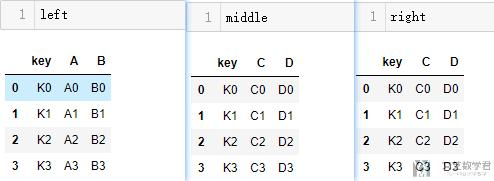1. functools.reduce(lambda x,y: pd.merge(x,y,on='key'),[left,middle,right])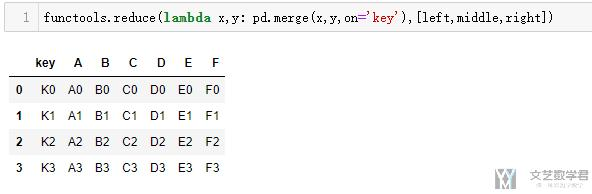### 数组的合并-join

1. df1 = pd.DataFrame(np.array([[1,2],[3,4],[5,6]]),columns=['a','b'])
2. df2 = pd.DataFrame(np.array([,,]),columns=['c'])
3. df1 = df1.join(df2)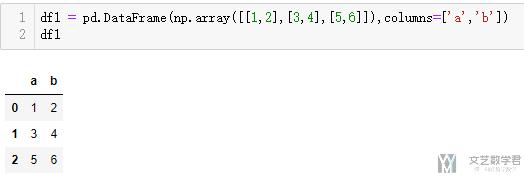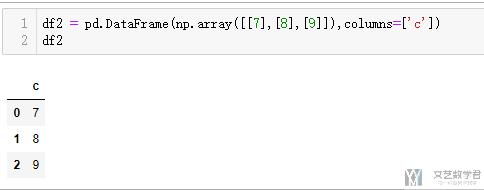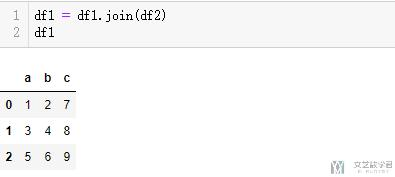## 分组-groupby操作

### groupby基础操作

1. df = DataFrame({'user_id': [5348, 13, 5348], 'course': [12, 45, 23], 'minutes': [9, 36, 45]})
2. >>
3.    course  minutes  user_id
4. 0      12        9     5348
5. 1      45       36       13
6. 2      23       45     5348

1. df['user_id']==5348
2. >>
3. 0     True
4. 1    False
5. 2     True
6. Name: user_id, dtype: bool
7. df[df['user_id']==5348]
8. >>
9.    course  minutes  user_id
10. 0      12        9     5348
11. 2      23       45     5348
12. df[df['user_id']==5348]['minutes'].sum()
13. >> 54

1. df.groupby('user_id').sum()
2. >>
3. user_id   course  minutes
4. 13             45       36
5. 5348         35       54

### 高级分组-对多个列同时进行分组与选择

1. df_mergedN = df_merged.groupby(['continent','date']).agg(dict_groupby)
2. df_mergedN.drop(['Country/Region', 'ISO3', 'Lat', 'Long'], axis=1, inplace=True)
3. df_mergedN1. df_mergedN[df_mergedN.index.get_level_values('continent') == 'Africa']

### 高级分组-不同列执行不同操作

2. A       1         3         10
3. A       1         1         12
4. B       2         3         10
5. B       1         2         20

1. Name    Sum1   Sum2    Average
2. A       2      4      11
3. B       3      5      15

1. df = (df.groupby('Name', as_index=False)

### 高级分组-不同列执行不同操作例子

1. dict_groupby = {i:'sumfor i in confirm.columns.values[4:]}
2. dict_groupby['Lat'] = 'mean'
3. dict_groupby['Long'] = 'mean'
4. dict_groupby

1. {...
2.  '5/9/20': 'sum',
3.  '5/10/20': 'sum',
4.  '5/11/20': 'sum',
5.  'Lat': 'mean',
6.  'Long': 'mean'}

1. # confirmed cases
2. confirmCountry = confirm.groupby('Country/Region').agg(dict_groupby)
3. # confirmCountry.drop('Cruise Ship', inplace=True)

## Map的使用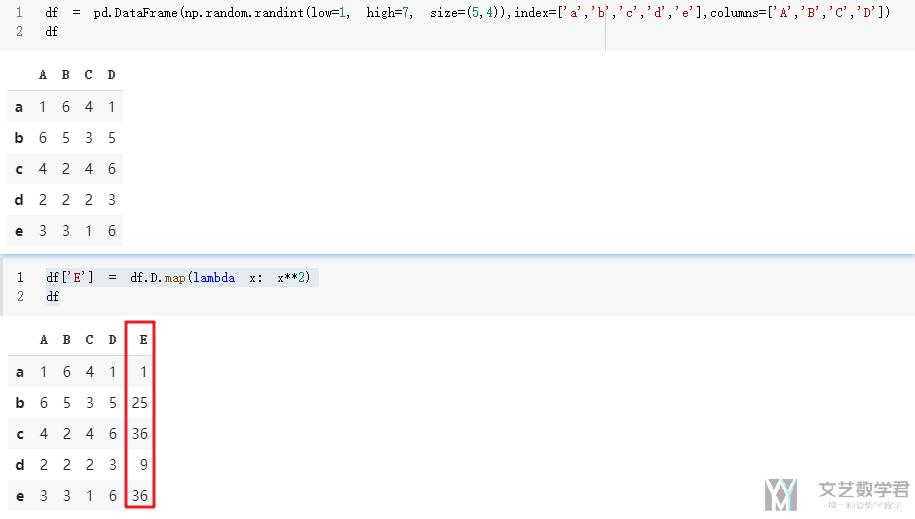### Apply与Transform的使用

1. df = pd.DataFrame(np.random.randint(low=0, high=10, size=(5,4)),index=['a','b','c','d','e'],columns=['A','B','C','D'])
2. df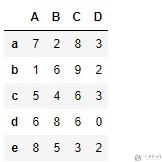1. df['E'] = df.apply(lambda x : x['A']+x['B']+x['C']+x['D'], axis=1)
2. df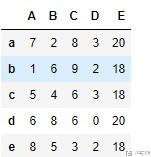1. # 这里是对E进行groupby, 计算一个group种D出现的次数
2. df['F'] = df.groupby('E')['D'].transform('nunique')
3. df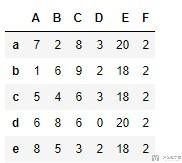## 文件的读取和保存

### 文件的读取

• index_col=0 : 把第一列作为index
• sep: 文件的分隔符

### pandas与numpy互相转换

• dataframe转换为numpy: df=df.values
• numpy转换为dataframe: df = pd.DataFrame(df)

## 时间数据处理

### 从str转换为datetime格式

2. a = pd.to_datetime(df['上班时间'], format="%H:%M:%S")
3. b = pd.to_datetime(df['下班时间'], format="%H:%M:%S")

1. df['minute'] = [times/pd.Timedelta('1 minute') for times in (b-a)]
2. df['hour'] = [times/pd.Timedelta('1 hour') for times in (b-a)]

### 时间序列的基本操作

``````from datetime import datetime

dates = [datetime(2018, 1, 1), datetime(2018, 1, 2), datetime(2018, 1, 3), datetime(2018, 1,4)]

ts = Series(np.random.randn(4), index=dates)

>>
2018-01-01   -1.959113
2018-01-02   -1.637479
2018-01-03    0.833776
2018-01-04    0.546243
dtype: float64``````

``````ts['2018/1/1']
>> -1.9591133192825554

ts['2018-1-1']
>> -1.9591133192825554``````

• start: 指定了日期范围的起始时间；
• end: 指定了日期范围的结束时间；
• periods: 指定了间隔范围，如果只是指定了`start``end`日期的其中一个，则需要改参数；
• freq: 指定了日期频率，比如`D`代表每天，`H`代表每小时，`M`代表月，这些频率字符前也可以指定一个整数，代表具体多少天，多少小时，比如`5D`代表`5`天。还有一些其他的频率字符串，比如`MS`代表每月第一天，`BM`代表每月最后一个工作日，或者是频率组合字符串，比如 `1h30min`代表`1 小时 30 分钟`
``````pd.date_range('2018','2019',freq='M')
>>
DatetimeIndex(['2018-01-31', '2018-02-28',                            '2018-03-31', '2018-04-30',
'2018-05-31', '2018-06-30', '2018-07-31', '2018-08-31',
'2018-09-30', '2018-10-31', '2018-11-30', '2018-12-31'],
dtype='datetime64[ns]', freq='M')``````

``````dates = pd.date_range('2018-1-1', '2018-1-2 23:00:00', freq='H')

ts = Series(np.arange(len(dates)), index=dates)

ts.size
>> 48``````

``````ts.resample('D').sum()
>>
2018-01-01    276
2018-01-02    852
Freq: D, dtype: int64``````

``````ts.resample('D').mean()
>>
2018-01-01    11.5
2018-01-02    35.5
Freq: D, dtype: float64``````

``````ts.resample('D').mean().resample('H').ffill()
>>
2018-01-01 00:00:00    11.5
2018-01-01 01:00:00    11.5
....
2018-01-01 20:00:00    11.5
2018-01-01 21:00:00    11.5
2018-01-01 22:00:00    11.5
2018-01-01 23:00:00    11.5
2018-01-02 00:00:00    35.5
Freq: H, dtype: float64``````

## Pandas 绘图

### 基础绘图

1. x = np.arange(-3, 3, 0.05)
2. y1 = x
3. y2 = x ** 2
4. y3 = np.sin(x)
5. data = pd.DataFrame(np.array([y1, y2, y3]).T, columns=['x', 'x^2', 'sin(x)'], index=x)
6. data.plot()
7. plt.show()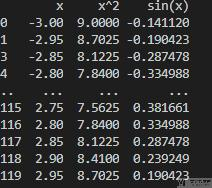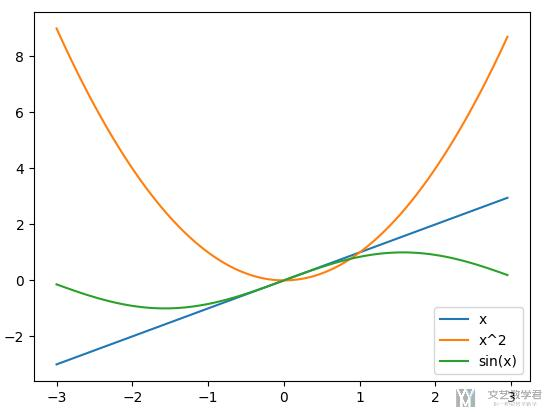### 设置标题，label等

1. matplotlib.style.use('fivethirtyeight') # bmh
4. ax.legend(bbox_to_anchor=(1.0, 0.9)) # 设置图例的位置
5. ax.set_title(u'不同基金服务号每篇的阅读量', fontsize=24, pad=30) # 设置标题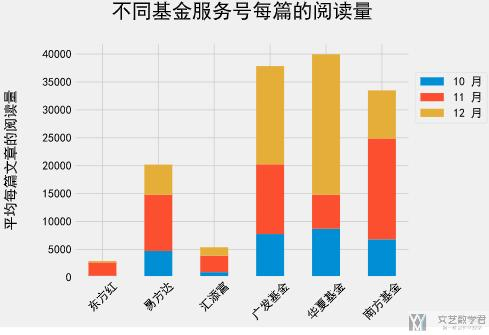### 绘制子图

1. fig, axs = plt.subplots(3, 1, figsize=(10, 12), facecolor='w', edgecolor='k', sharex=True)
2. data.iloc[:,0].plot(kind='line', ax=axs)
3. data.iloc[:,1].plot(kind='line', ax=axs)
4. data.iloc[:,2].plot(kind='line', ax=axs)
5. plt.show()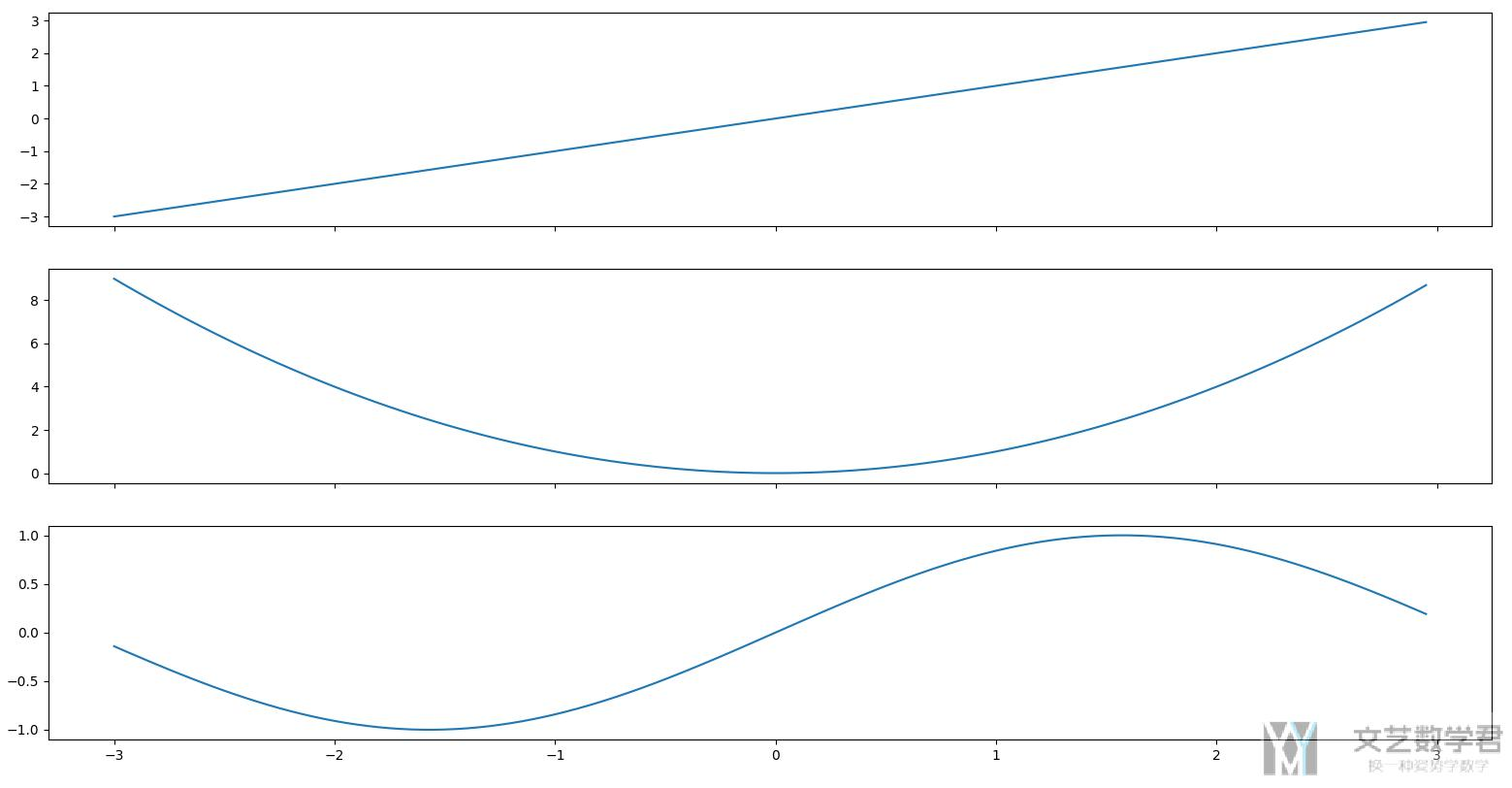### 设置 legend

1. fig, axs = plt.subplots(3, 1, figsize=(10, 12), facecolor='w', edgecolor='k', sharex=True)
2. data.iloc[:,0].plot(kind='line', ax=axs, legend=True)
3. data.iloc[:,1].plot(kind='line', ax=axs, legend=True)
4. data.iloc[:,2].plot(kind='line', ax=axs, legend=True)
5. plt.show()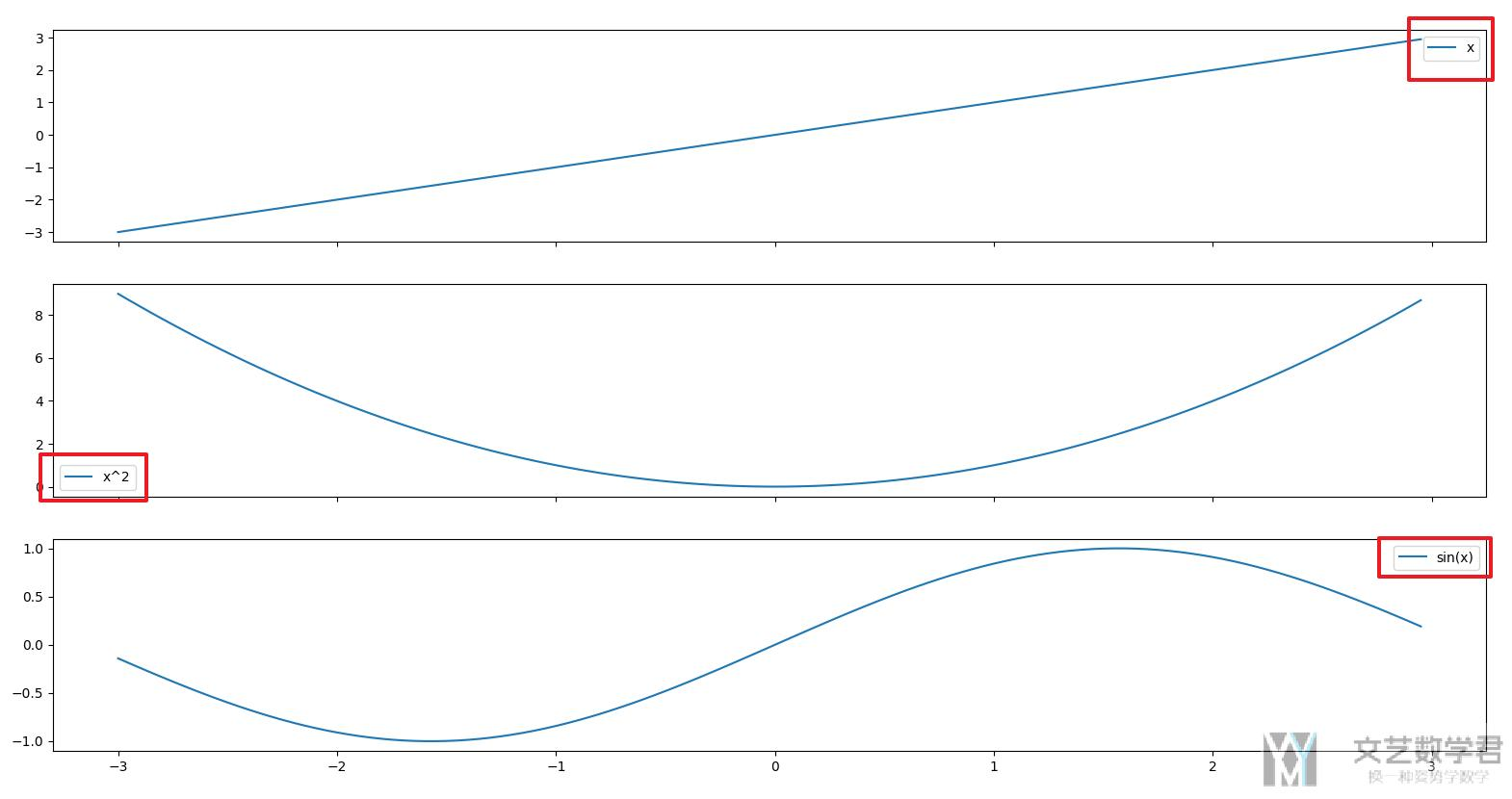### 调整 xticks 的大小和角度

1. data.iloc[:,0].plot(kind='line', ax=axs, legend=True, rot=60, fontsize=23)
2. data.iloc[:,1].plot(kind='line', ax=axs, legend=True, rot=60, fontsize=23)
3. data.iloc[:,2].plot(kind='line', ax=axs, legend=True, rot=60, fontsize=23)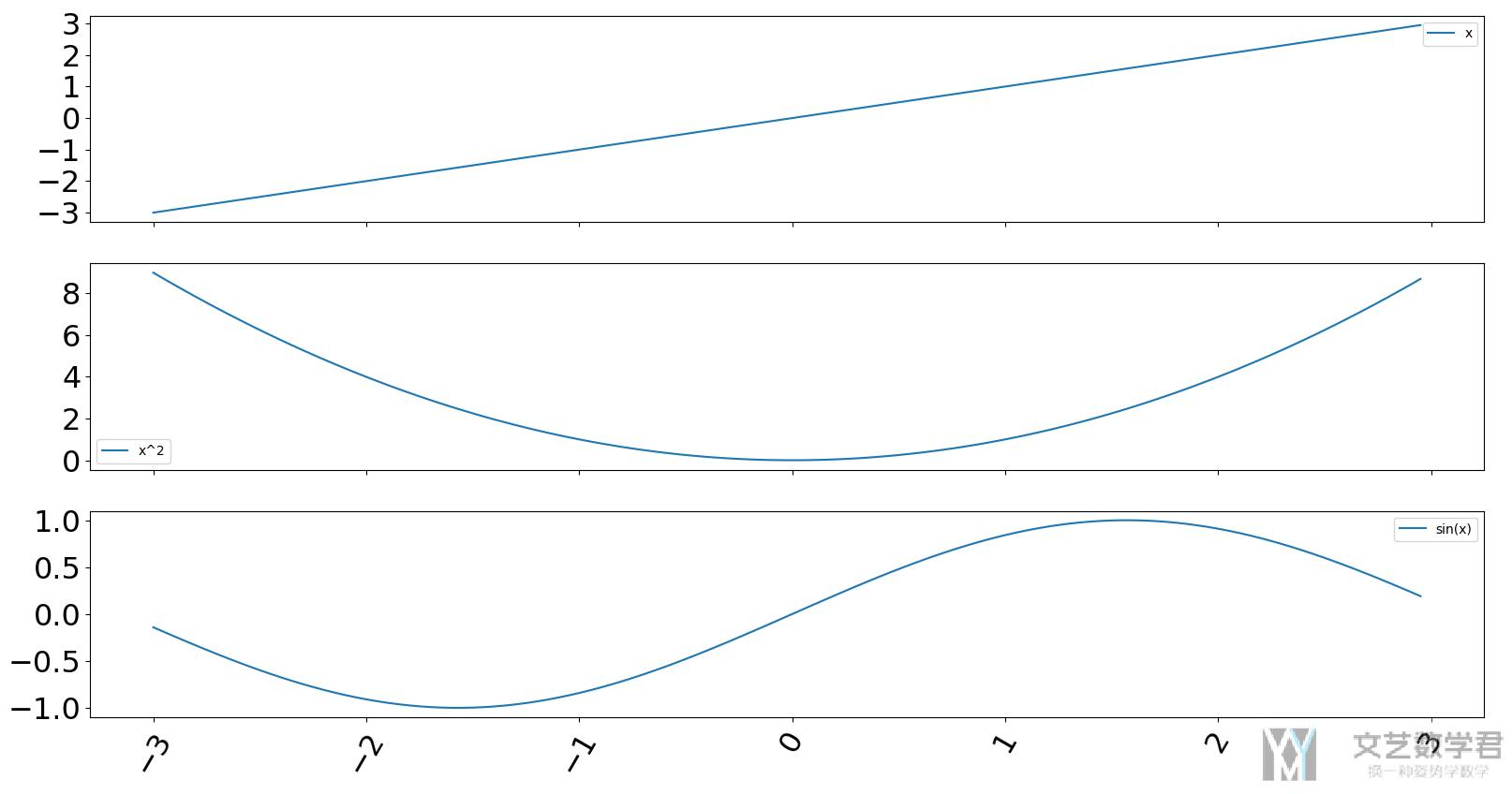### 调整 xticks 的内容

1. fig, axs = plt.subplots(3, 1, figsize=(10, 12), facecolor='w', edgecolor='k', sharex=True)
2. data.iloc[:,0].plot(kind='line', ax=axs, legend=True, rot=60, fontsize=23, yticks=[1,2], xticks=[-2.5, -1.5, 0, 1.5, 2.5])
3. data.iloc[:,1].plot(kind='line', ax=axs, legend=True, rot=60, fontsize=23)
4. data.iloc[:,2].plot(kind='line', ax=axs, legend=True, rot=60, fontsize=23)
5. plt.show()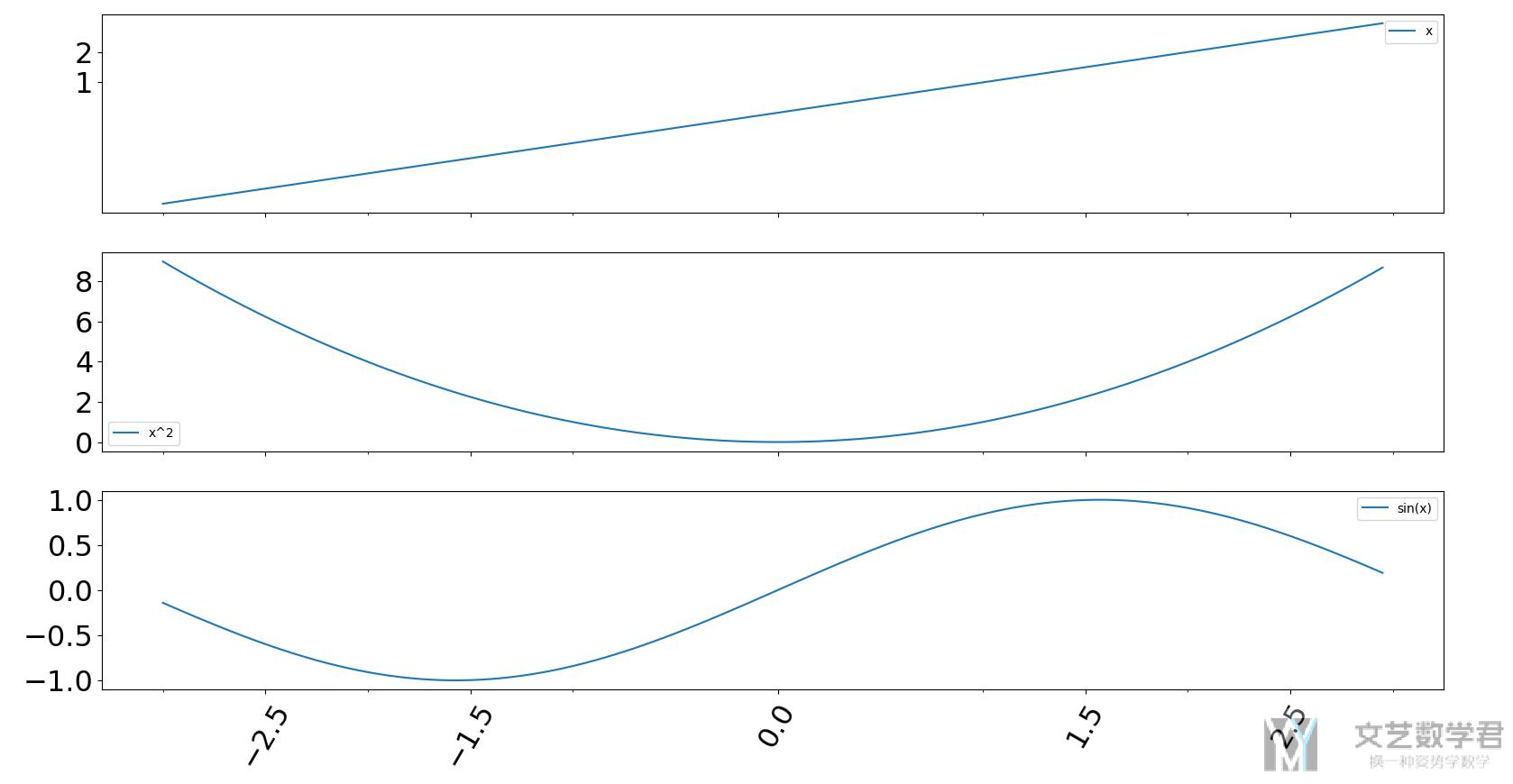• 微信公众号
• 关注微信公众号
•• QQ群
• 我们的QQ群号
•• 本文由 发表于 2018年4月27日06:04:32
• 转载请务必保留本文链接：https://mathpretty.com/9258.html